Next: Contrasts Up: tr01cb1 Previous: Model Equivalence

# Confounds

The previous section assumed that all the first-level parameter estimates would be used in the second-level model. This is often not true, as parameters of no interest (confounds) are often present in the first-level to remove unwanted signals (e.g. motion estimates as regressors to remove motion-related artefacts). This section shows that, when confounds are present in the first level, the model equivalence theorem still holds. That is, the second-level (group) analysis does not need to know about the confounds defined in the first level and only requires the estimates and covariance estimates of the parameters of interest. The proof of this result follows.

Consider a first-level GLM including confounds. This model can be written as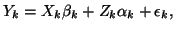where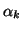are the parameters of no interest (confounds) and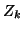contains the regressors of no interest. Combining across subjects but grouping the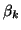andseparately gives the model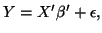whereand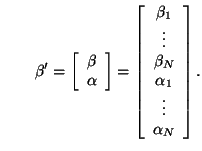The parameter estimates in this case are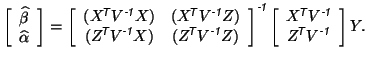It is always possible to re-write such a model so that the confounds are orthogonal to the signals of interest in the pre-whitened space, without affecting the estimates of the signals of interest. This result is formally stated and proven in the appendix as Theorem A. Therefore, we can impose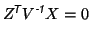(12)

which gives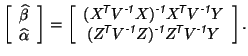(13)

In the second-level, only the parameters of interest,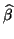, appear. That is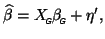which is identical to equation 6, so that the parameter estimates are exactly the same as those given in equation 7.

On the other hand, the single-level model with confounds is written as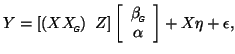which has the same noise terms and covariance structure as before. The parameter estimates are given by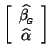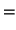Using equation 11 from the previous section, together with the orthogonality condition in equation 12, it is easy to show that: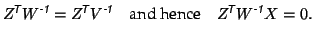This gives the parameter estimates as: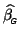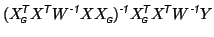(14)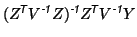(15)

where equation 14 is the same as equation 8, and consequently, using the previous theorem, equivalent to the two-level estimation when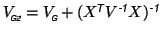, while equation 15 is precisely the same as the two-level version (equation 13) independent of the choice of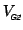. The variances of the parameter estimates are also equal under these conditions, which can be verified by straightforward calculations.

Therefore the model equivalence result still holds when confounds are present.Next: Contrasts Up: tr01cb1 Previous: Model Equivalence
Christian Beckmann 2003-07-16